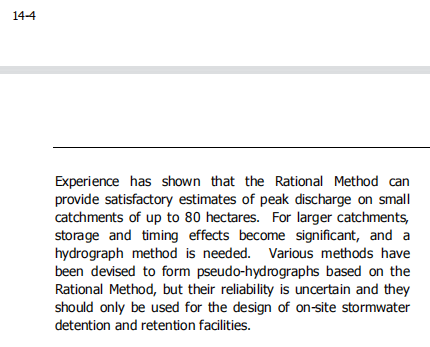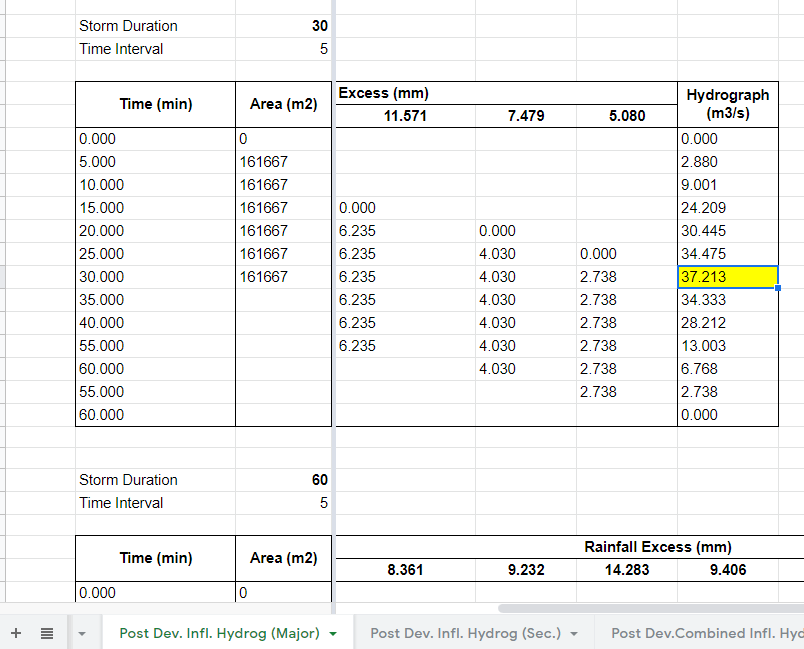603 - 5885 1250 (4 lines) info@mes100.com
Select Page

# Introduction#

There are two basic methods to computing detention pond flow, which are Rational Method and Time Area method. According to MSMA 1st Edition Chapter 14, Rational Method is recommended on small catchments up to 80 hectares.“Rational Method can provided satisfactory estimates of peak discharge on small catchments of up to 80 hectares”

Time Area method is recommended for a big area which is bigger than 80 hectares and used whenever temporal variations effects need to be considered. This method assumes that the outflow is characterized by separable subcatchments.

This article will explain more on the steps used in Time Area Method and a benchmark conjunction to MSMA 2nd Edition Chapter 2 Appendix 2.F2 Time Area Hydrograph Method.

However, some of the calculations are not available in MSMA and we added it in order to adapt it in MiTS, such as Minor and Secondary ARI, and includes the outlet control calculation, such as stage storage relationship, stage discharge relationship, preliminary storage volume and pool routing.

# Example : Given Information#

Attachments:

## Summary Information#

ARI : 20 year

Development Area : 97 Hectare

Development Area : Wangsa Maju, Kuala Lumpur

Raingauge Location : Ibu Pejabat JPS, Kuala Lumpur

# General Procedures#

## Setting grid system#

The development area is defined by grid in order to calculate the Time of Concentration (tc). Example of grid is as shown in Figure 2.F4

## Estimate overland flow time, to and drain time, td to calculate the tc. #

Estimate the to and td towards the outlet and sum up the values to calculate tc. Detailed calculation is as in Table 2.F4.

Estimate to by using Horton Equation.

Estimate td by using Manning Equation

However, flow velocity is assumed to be 1 m/s for the sake of simplicity.

### How to adapt the values into MiTS#

Plug in the value for td and to based on the longest tc, or simply override the tc value in the tc box.

## Plot contour of equal tc interval to produce isochrones#

The isochrones are produced by plotting the contour with equal tc (5, 10, 15, 20, 25 minutes…) which has been calculated in the previous calculation.

## Estimate area between isochrones#

The area for sub catchments is calculated assisted by AutoCAD. Example of the sub catchment area based on the isochrones is as in the table below.

### How to adapt in MiTS#

The number of blocks or number of sub catchments are determined by tc and region. Since the development area is located in Kuala Lumpur region, therefore Appendix 2.C5 Region 5 is referred to.

Based on the appendix, the number of blocks of 30 minute is 6, therefore 6 subcatchments are divided (A1 – A6). Default areas of each of the subcatchments are divided equally, which is approximately 16.167 ha for each sub catchment.

## Calculate Rainfall Intensity#

Use MSMA 2 Equation 2.2 to calculate Rainfall Intensity

The calculation of rainfall intensity in MSMA is based on 20 years ARI.

Pre development and post development for all ARI detailed calculations can be referred to these spreadsheets.

## Calculate Rainfall Excess (RE)#

Total Rainfall is deducted by losses, to calculate the Rainfall Excess (RE). The equation of Rainfall Excess is:

RE = Rainfall Temporal Pattern – Losses

By using Appendix 2.C5, rainfall temporal pattern is obtained from 30-min duration.

The result in MSMA for the rainfall temporal pattern is benchmarked based on 20 years ARI for impervious catchment conditions at 30 minutes duration.

Given the calculated total rainfall and temporal pattern, RE is calculated. Rainfall losses for the design storm of pervious and impervious is deducted based on in Table 2.6.

There is a small discrepancy in terms of calculation of losses between MiTS and MSMA due to different methods of calculating the RE. Therefore, the inflow hydrograph in the later section will have a discrepancy due to the losses. Detailed calculation of pre and post development RE is as in the spreadsheet below. The calculation comprises pre and post development with pervious and impervious catchment conditions at different storm duration.

## Calculate the hydrograph ordinate#

Estimate the runoff hydrograph and peak flow rates. The example below is based on major storm design with impervious catchment. Note that the calculation will be different from MSMA due to different areas of subcatchment and different losses calculation.

Use Equation 2.5 to calculate the inflow flow rate.Manual calculation Post development peak flow rate is 37.213 m3/s at 30 min for major ARIMiTS textual report Post development peak flow rate is 37.213 m3/s at 30 min for major ARI

Other calculations of other ARIs are calculated and attached as per link below.

This section onwards is not available in MSMA which is related to final design of a detention pond which involves preliminary pond volume, stage storage, stage discharge and pool routing to calculate the maximum discharge and maximum water level for each ARI. The benchmark can only be done between MiTS and the manual calculation.

## Compute the preliminary volume#

Before stage storage and stage discharge can be developed, a preliminary estimation of pond sizing is required. This stage shall be done for a major system which is at 20 years ARI. Preliminary volume is a judgement reference to determine the initial storage.

The equation used in calculating the estimated storage volume is referred to MSMA 1 Equation 20.13.

The preliminary pond volume shown in MiTS input in the Pond Sizing tab is 26167.864 m3.

Detailed manual calculation can be obtained from the “Preliminary Volume” spreadsheet. It shows a detailed calculation for each of the storm duration. The estimated volume required is 26168 m3 at 30-min storm duration.

## Compute Stage Storage relationship#

Stage storage relationship is used to determine the initial storage volume. Use the double-end area formula to compute the provided storage volume.

## Compute Stage Discharge Relationship#

Calculate the stage discharge relationship from minor ARI and build up the equation to major and secondary ARI storm design. The stage storage relationship will be used later to plot the inflow hydrograph routing. See “Max Q&WL” spreadsheet for stage discharge calculation.

Equation 2.6 MSMA 2 is used to calculate the orifice outlet discharge for minor and major ARI design storm.

Equation 2.10 MSMA 2 is used to calculate the broad crested weir for secondary ARI design storms.

The coefficient for the weir is based on MSMA 1 Design Chart 20.1 which is plotted between weir base width and the head.

The stage discharge relationship in MiTS textual report will be shown right under the inflow hydrograph routing respective to its own ARI design storm.

## Determine the maximum outlet discharge and maximum water level#

The maximum discharge and maximum water level can be obtained by using Modified Puls Method MSMA 2 Equation 2.17.

You can refer to the inflow hydrograph routing for minor, major and secondary ARI in the “Max Q&WL” spreadsheet. The stage discharge relationship is combined in a page so that it is easier to plot the interpolation.

Summary of Qmax and WLmax also can be found quickly right under the secondary outlet in Outlet Design Input tab without analysing.